Browse by Topic

# New in the Wolfram Language: Symbolic PDEs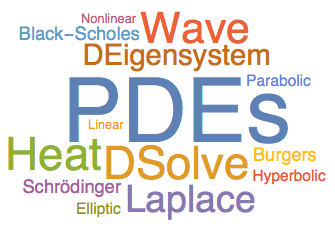Partial differential equations (PDEs) play a vital role in mathematics and its applications. They can be used to model real-world phenomena such as the vibrations of a stretched string, the flow of heat in a bar, or the change in values of financial options. My aim in writing this post is to give you a brief glimpse into the fascinating world of PDEs using the improvements for boundary value problems in DSolve and the new DEigensystem function in Version 10.3 of the Wolfram Language.

The history of PDEs goes back to the works of famous eighteenth-century mathematicians such as Euler, d’Alembert, and Laplace, but the development of this field has continued unabated during the last three centuries. I have, therefore, chosen examples of both classical as well as modern PDEs in order to give you a taste of this vast and beautiful subject.

Let us begin by considering the vibrations of a stretched string of length π that is fixed at both ends. The vibrations of the string can be modeled by the one-dimensional wave equation, which is given below. Here, u(x,t) is the vertical displacement of a point at the location x on the string, at time t: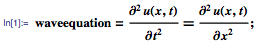Next, we specify a boundary condition to indicate that the ends of the string remain fixed during the vibrations: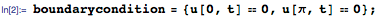Finally, we prescribe the displacement and speed at different points on the string at time t=0 as initial conditions for the motion of the string:We can now use DSolve to solve the initial boundary value problem for the wave equation: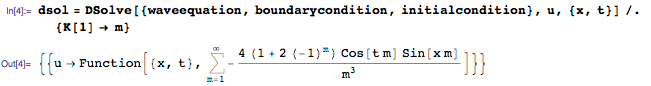As seen above, the solution is an infinite sum of trigonometric functions. The sum is returned in an Inactive form since each individual term in the expansion has a physical interpretation and, typically, a small number of terms will provide a useful approximation to the exact solution. For example, we can extract four terms from the sum to obtain an approximate solution asol(x,t):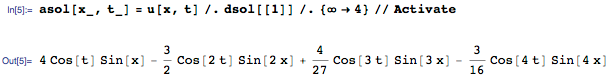Each term in the sum represents a standing wave, which can be visualized as follows: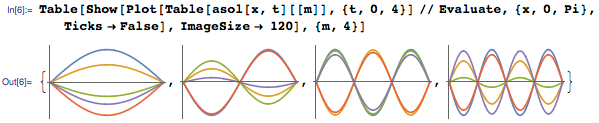These standing waves combine in a magical way to produce the smooth vibrations of the string, as shown in the following animation: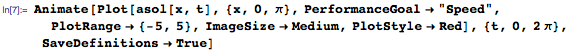The wave equation belongs to the class of linear hyperbolic PDEs, which govern the propagation of signals with finite speeds. This PDE arose as a convenient way to model the vibrations of strings and other deformable bodies, but it plays an even more important role in modern physics and engineering since it governs the propagation of light and other electromagnetic waves.

Let us now model the flow of heat in a bar of length 1 that is insulated at both ends using the heat equation, which is given as follows: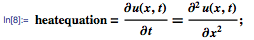Since the bar is insulated at both ends, no heat flows through the ends, which translates into a boundary condition at the two ends x=0 and x=1: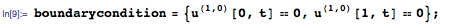Next, we must prescribe the initial temperature at different points in the bar. In this example, we choose the linear function given below. The initial temperature is 20 at the left end (x=0) and 100 at the right end (x=1):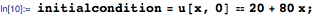Finally, we solve the heat equation subject to these conditions: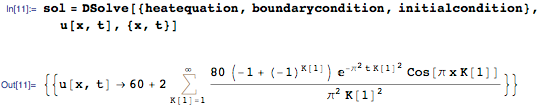As in the case of the wave equation, we can extract a few terms from the Inactive sum to obtain an approximate solution, as shown below: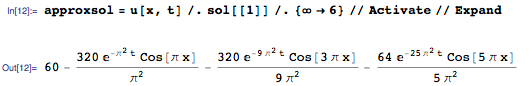The first term in the approximate solution, 60, is the average of the initial temperatures at the left and the right ends of the bar, and is also the steady-state temperature for this bar. As the plot of temperature along the length of the bar below shows, the temperature of the bar quickly evolves to the steady-state value of 60 degrees: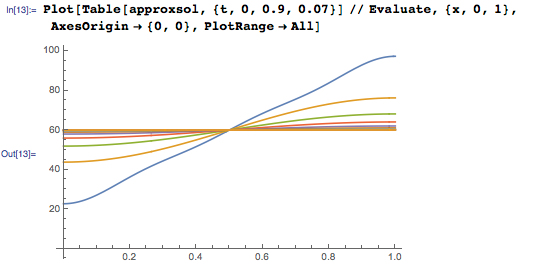The heat equation belongs to the class of linear parabolic PDEs, which govern diffusion processes. This rather simple-looking equation is ubiquitous and makes a surprising appearance in a wide variety of applications. We will see two examples of this phenomenon later in the post.

We now turn to the Laplace equation, which is used to model the steady state of systems, i.e. the behavior after any time-dependent transients have died out. In two dimensions, this equation is given as follows: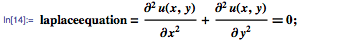Assume that the coordinates x and y are restricted to the rectangular region Ω given below: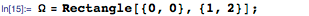The classical Dirichlet problem is to find a function u(x,y) that satisfies the Laplace equation inside Ω along with a given DirichletCondition that specifies values on the boundary of Ω, as illustrated below: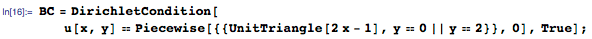The Dirichlet problem can be solved using the elegant region notation for DSolve: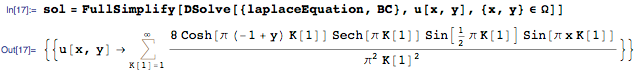As in earlier examples, we can extract a fixed number, say 100, of terms from the Inactive sum and visualize the solution, as shown below: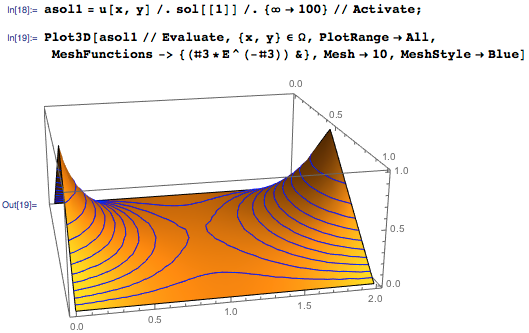Notice that the solution u(x,y) of the Dirichlet problem is smooth within Ω despite the fact that the boundary conditions have sharp features. Also, u(x,y) attains its maximum and minimum values on the boundary, while there is a saddle point at the center of the rectangle. These features are typical of linear elliptic equations, the class of PDEs to which the Laplace equation belongs.

The wave, heat, and Laplace equations are the most famous examples of classical PDEs. We will now consider three representative examples of modern PDEs, beginning with Burgers’ equation for viscous fluid flow, which can be stated as follows: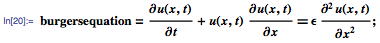This nonlinear PDE was introduced by J. M. Burgers around 1940 as a simple model for turbulent flows (the parameter ϵ in the equation represents the viscosity of the fluid). However, a decade later, E. Hopf and J. D. Cole showed that Burgers’ equation can be transformed into the heat equation, thereby indicating that this equation cannot exhibit chaotic behavior. The Hopf–Cole transformation allows us to solve Burgers’ equation in closed form for an initial condition, such as the one given below: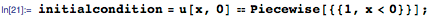For this example, we use DSolveValue, which returns only an expression for the solution. The error function (Erf) terms in the formula below arise from the solution of the corresponding initial value problem for the heat equation: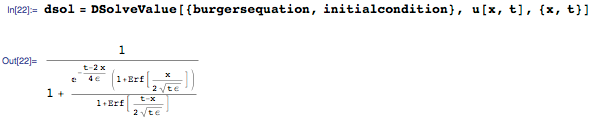The following plot is showing the evolution over time of a hypothetical fluid velocity field in one dimension. The solution is smooth for a positive value of ϵ, although the initial condition is a piecewise function: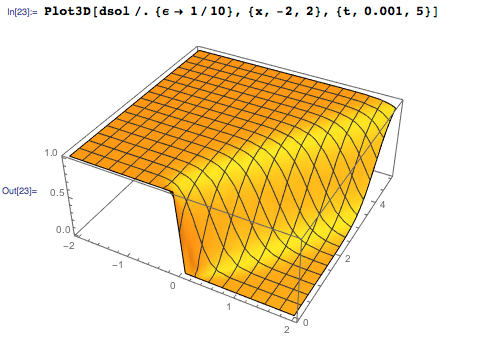As seen below, the solution develops a shock discontinuity in the limit when the viscosity ϵ approaches 0. These shock solutions are a well-known feature of the inviscid (ϵ = 0) Burgers’ equation: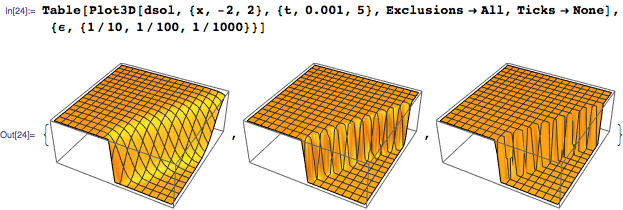As a second example of a modern PDE, let us consider the Black–Scholes PDE from finance. This equation was introduced by Fischer Black and Myron Scholes in 1973 as a model for the prices of European-style financial options, and can be stated as follows: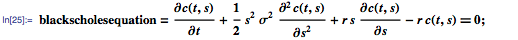Here, c is the price of the option as a function of stock price s and time t, r is the risk-free interest rate, and σ is the volatility of the stock.

In their landmark paper (which has been cited more than 28,000 times), Black and Scholes noted that their equation can be reduced to the heat equation by a transformation of variables. This dramatic simplification leads to the celebrated Black–Scholes formula for European call options, under a terminal condition based on the “strike price” k of the asset at time t=T: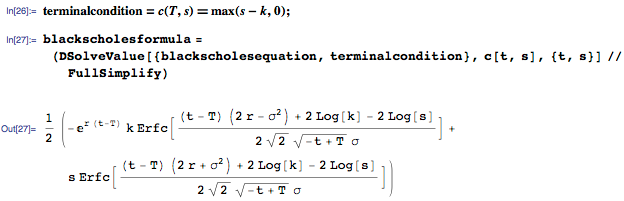Armed with this formula, we can compute the value of a financial option for typical values of the parameters: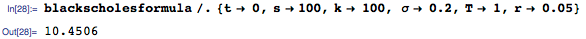The answer agrees with the value given by the built-in FinancialDerivative function: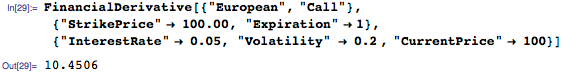As our third example of a modern PDE, we will solve the free Schrödinger equation for an electron that is constrained to move in a one-dimensional box of length d, with a suitable initial condition. The equation and conditions may be stated as follows: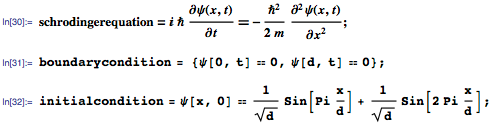This example has an elementary solution that takes imaginary values due to the presence of I in the Schrödinger equation: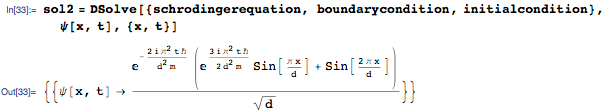The probability density function for the electron, ρ=Ψ Ψ, can be computed using suitable values for the parameters in the problem as follows: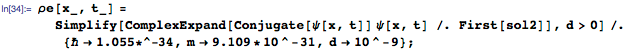We can create a movie showing the evolution of the probability density over time, which reveals that the “center” of the electron moves from side to side in the box:Eigenvalues and eigenfunctions play an important role in the solution of Schrödinger’s equation and other PDEs. In particular, they provide the building blocks for the infinite sum solutions of the wave and heat equations that we saw earlier in the post. Hence, as our final example, let us consider the problem of finding the nine smallest eigenvalues and eigenfunctions for the Laplacian operator with a homogeneous (zero) Dirichlet condition over a ball in three dimensions. That is, we are interested in the nine smallest values of λ and the corresponding functions ϕ that satisfy 𝓛ϕ = λ ϕ, with the following definitions: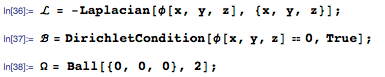The new DEigensystem function in Version 10.3 allows us to compute the required eigenvalues and eigenfunctions as follows: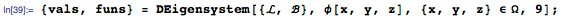The eigenvalues for this problem are expressed in terms of BesselJZero. For example: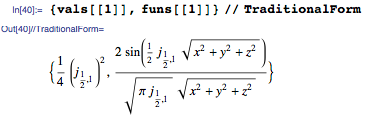The eigenfunctions can be visualized using DensityPlot3D, which returns the beautiful plots that are shown below: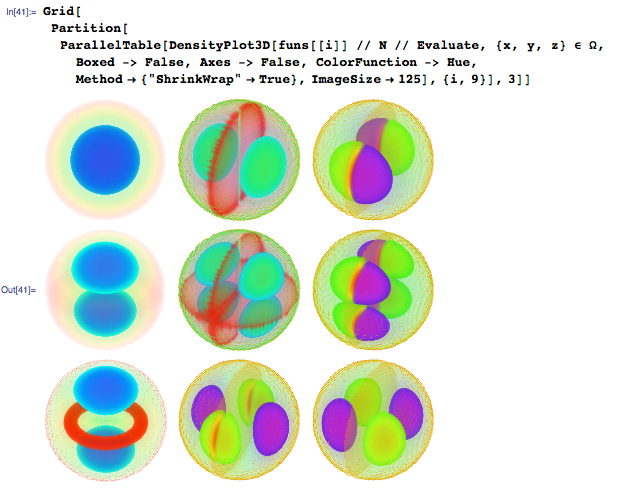Partial differential equations are an essential tool in many branches of science, engineering, statistics, and finance. At a more fundamental level, they provide precise mathematical formulations for some of the deepest and most subtle questions about our universe, such as whether naked singularities can really exist. In my experience, the study of PDEs rewards one with a rare combination of practical insights and intellectual satisfaction.

I invite you to look at the documentation for DSolve, NDSolve, DEigensystem, NDEigensystem, and the finite element method to learn more about the various approaches for solving PDEs in the Wolfram Language.

Symbolic PDEs is supported in Version 10.3 of the Wolfram Language and Mathematica, and is rolling out soon in all other Wolfram products.